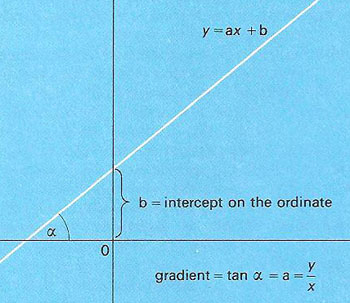A# linearThe graph of y = ax + b.

To be linear implies having only a multiplicative factor. A linear relationship is a relationship between two variables such that, if one is plotted as a function of the other (see analytical geometry), the result is a straight line. If f( x) is a linear function, then f (a+b) = f (a) + f (b) and c f (x) = f (cx) must both be true for all values of a, b, c, and x.